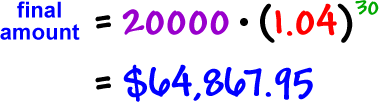Let's try something messier:

If we invest \$20,000 in an account that pays 8% compounded semi-annually, how much will be in the account in 15 years?

initial amount = 20,000

At the end of each period (six months), we'll be earning 4%...

So, each \$1.00 will turn into \$1.04...

growth factor = \$1.04

number of periods = 30
(That's twice a year for 15 years.)(I rounded it to two places, since it's money.)

TRY IT:

You invest \$60,000 in an account that pays 9% compounded semi-annually.  How much will you have in your account in 25 years?